# 2 Prerequisits: Basic statistical terms

This chapter introduces some important terms useful for doing data analyses. It also introduces the essentials of the classical frequentist tests such as t- and Chisquare test. Even though we will not use nullhypotheses tests later , we introduce them here because we need to understand 100 years of scientific literature. For each classical test, we provide a suggestion how to do it in a Bayesian way and we discuss some differences between the Bayesian and frequentist statistics.

## 2.1 Variables and observations

Empirical research involves data collection. Data are collected by recording measurements of variables for observational units. An observational unit may be, for example, an individual, a plot, a time interval or a combination of those. The collection of all units ideally build a random sample of the entire population of units in that we are interested. The measurements (or observations) of the random sample are stored in a data table (sometimes also called data set, but a data set may include several data tables. A collection of data tables belonging to the same study or system is normally bundled and stored in a data base). A data table is a collection of variables (columns). Data tables normally are handled as objects of class data.frame in R. All measurements on a row in a data table belong to the same observational unit. The variables can be of different scales (Table 2.1).

Table 2.1: Scales of measurements
Scale Examples Properties Coding in R
Nominal Sex, genotype, habitat Identity (values have a unique meaning) factor()
Ordinal Elevational zones Identity and magnitude (values have an ordered relationship) ordered()
Numeric Discrete: counts; continuous: body weight, wing length Identity, magnitude, and intervals or ratios intgeger() numeric()

## 2.2 Displaying and summarizing variables

While nominal and ordinal variables are summarized by giving the absolute number or the proportion of observations for each category, numeric variables normally are summarized by a location and a scatter statistics, such as the mean and the standard deviation or the median and some quantiles. The distribution of a numeric variable is graphically displayed in a histogram (Fig. 2.1).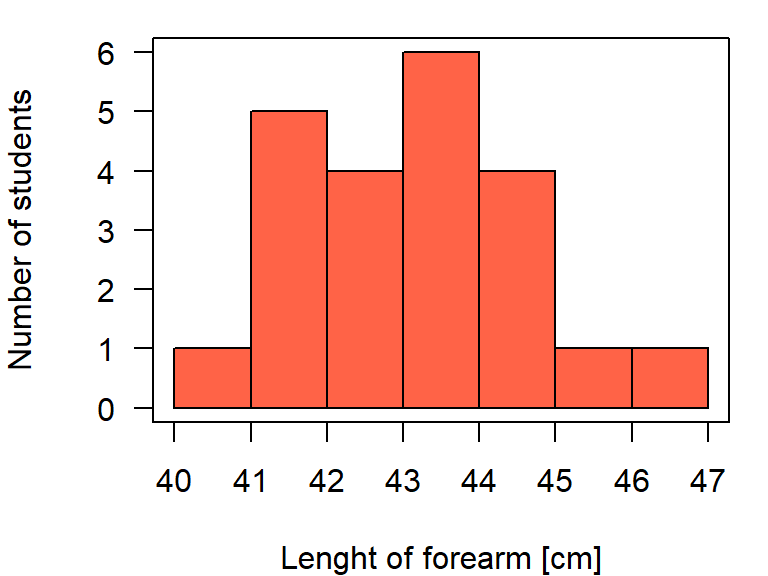Figure 2.1: Histogram of the length of ell of statistics course participants.

To draw a histogram, the variable is displayed on the x-axis and the $$x_i$$-values are assigned to classes. The edges of the classes are called ‘breaks.’ They can be set with the argument breaks= within the function hist. The values given in the breaks= argument must at least span the values of the variable. If the argument breaks= is not specified, R searches for breaks-values that make the histogram look smooth. The number of observations falling in each class is given on the y-axis. The y-axis can be re-scaled so that the area of the histogram equals 1 by setting the argument density=TRUE. In that case, the values on the y-axis correspond to the density values of a probability distribution (Chapter 4).

Location statistics are mean, median or mode. A common mean is the - arithmetic mean: $$\hat{\mu} = \bar{x} = \frac{i=1}{n} x_i \sum_{1}^{n}$$(R function mean), where $$n$$ is the sample size. The parameter $$\mu$$ is the (unknown) true mean of the entire population of which the $$1,...,n$$ measurements are a random sample of. $$\bar{x}$$ is called the sample mean and used as an estimate for $$\mu$$. The $$^$$ above any parameter indicates that the parameter value is obtained from a sample and, therefore, it may be different from the true value.

The median is the 50% quantile. We find 50% of the measurements below and 50% above the median. If $$x_1,..., x_n$$ are the ordered measurements of a variable, then the median is: - median $$= x_{(n+1)/2}$$ for uneven $$n$$, and median $$= \frac{1}{2}(x_{n/2} + x_{n/2+1})$$ for even $$n$$ (R function median).

The mode is the value that is occurring with highest frequency or that has the highest density.

Scatter also is called spread, scale or variance. Variance parameters describe how far away from the location parameter single observations can be found, or how the measurements are scattered around their mean. The variance is defined as the average squared difference between the observations and the mean:

• variance $$\hat{\sigma^2} = s^2 = \frac{1}{n-1}\sum_{i=1}^{n}(x_i-\bar{x})^2$$
The term $$(n-1)$$ is called the degrees of freedom. It is used in the denominator of the variance formula instead of $$n$$ to prevent underestimating the variance. Because $$\bar{x}$$ is in average closer to $$x_i$$ than the unknown true mean $$\mu$$ would be, the variance would be underestimated if $$n$$ is used in the denominator.

The maximum likelihood estimate (Chapter xxx.xx) of the variance corresponds to the variance formula using $$n$$ instead of $$n-1$$ in the denominator, see, e.g., .

The variance is used to compare the degree of scatter among different groups. However, its values are difficult to interpret because of the squared unit. Therefore, the square root of the variance, the standard deviation is normally reported:

• standard deviation $$\hat{\sigma} = s = \sqrt{s^2}$$ (R Function sd)

The standard deviation is approximately the average deviation of an observation from the sample mean. In the case of a normal distribution, about two thirds (68%) of the data are expected within one standard deviation around the mean.

The variance and standard deviation each describe the scatter with a single value. Thus, we have to assume that the observations are scattered symmetrically around their mean in order to get a picture of the distribution of the measurements. When the measurements are spread asymmetrically (skewed distribution), then it may be more precise to describe the scatter with more than one value. Such statistics could be quantiles from the lower and upper tail of the data.

Quantiles inform us about both location and spread of a distribution. The $$p$$th-quantile is the value with the property that a proportion $$p$$ of all values are less than or equal to the value of the quantile. The median is the 50% quantile.The 25% quantile and the 75% quantile are also called the lower and upper quartiles, respectively. The range between the 25% and the 75% quartile is called the interquartile range. This range includes 50% of the distribution and is also used as a measure of scatter. The R function quantile extracts sample quantiles. The median, the quartiles, and the interquartile range can be graphically displayed using box and-whisker plots (boxplots in short, R function boxplot). The horizontal fat bars are the medians (Fig. 2.2). The boxes mark the interquartile range. The whiskers reach out to the last observation within 1.5 times the interquartile range from the quartile. Circles mark observations beyond 1.5 times the interquartile range from the quartile.

par(mar=c(5,4,1,1))
boxplot(ell~car, data=dat, las=1, ylab="Lenght of ell [cm]",
col="tomato", xaxt="n")
axis(1, at=c(1,2), labels=c("Not owing a car", "Car owner"))
n <- table(dat$car) axis(1, at=c(1,2), labels=paste("n=", n, sep=""), mgp=c(3,2, 0))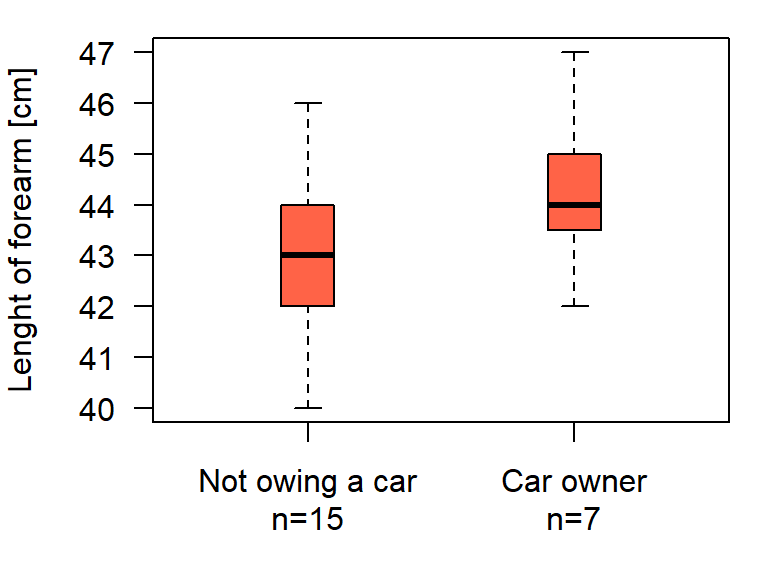Figure 2.2: Boxplot of the length of ell of statistics course participants who are or ar not owner of a car. The boxplot is an appealing tool for comparing location, variance and distribution of measurements among groups. ### 2.2.1 Correlations A correlation measures the strength with which characteristics of two variables are associated with each other (co-occur). If both variables are numeric, we can visualize the correlation using a scatterplot. par(mar=c(5,4,1,1)) plot(temp~ell, data=dat, las=1, xlab="Lenght of ell [cm]", ylab="Comfort temperature [°C]", pch=16)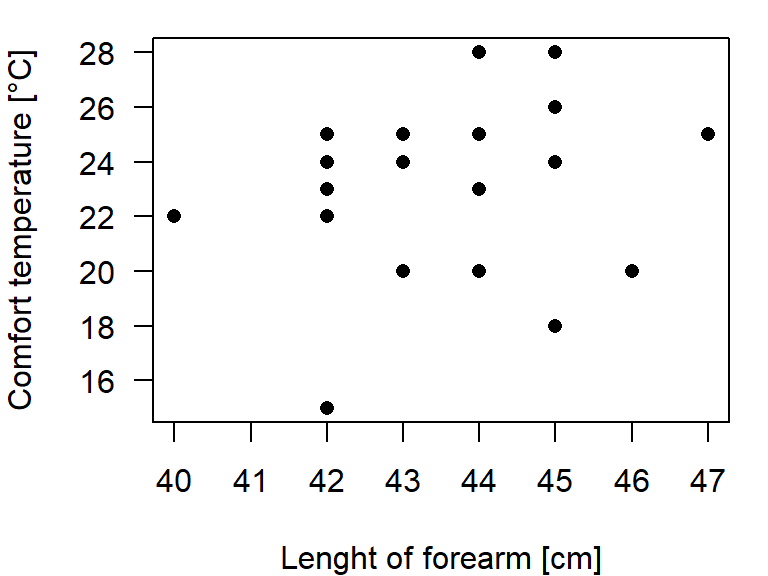Figure 2.3: Scatterplot of the length of ell and the comfort temperature of statistics course participants. The covariance between variable $$x$$ and $$y$$ is defined as: • covariance $$q = \frac{1}{n-1}\sum_{i=1}^{n}((x_i-\bar{x})*(y_i-\bar{y}))$$ (R function cov) As for the variance, also the units of the covariance are sqared and therefore covariance values are difficult to interpret. A standardized covariance is the Pearson correlation coefficient: • Pearson correlation coefficient: $$r=\frac{\sum_{i=1}^{n}(x_i-\bar{x})(y_i-\bar{y})}{\sqrt{\sum_{i=1}^{n}(x_i-\bar{x})^2\sum_{i=1}^{n}(y_i-\bar{y})^2}}$$ (R function cor) Means, variances, standard deviations, covariances and correlations are sensible for outliers. Single observations containing extreme values normally have a overproportional influence on these statistics. When outliers are present in the data, we may prefer a more robust correlation measure such as the Spearman correlation or Kendall’s tau. Both are based on the ranks of the measurements instead of the measurements themselves. • Spearman correlation coefficient: correlation between rank(x) and rank(y) (R function cor(x,y, method="spearman")) • Kendall’s tau: $$\tau = 1-\frac{4I}{(n(n-1))}$$, where $$I$$ = number of pairs $$(i,k)$$ for which $$(x_i < x_k)$$ & $$(y_i > y_k)$$ or viceversa. (R function cor(x,y, method="kendall")) ### 2.2.2 Principal components analyses PCA The principal components analysis (PCA) is a multivariate correlation analysis. A multidimensional data set with $$k$$ variables can be seen as a cloud of points (observations) in a $$k$$-dimensional space. Imagine, we could move around in the space and look at the cloud from different locations. From some locations, the data looks highly correlated, whereas from others, we cannot see the correlation. That is what PCA is doing. It is rotating the coordinate system (defined by the original variables) of the data cloud so that the correlations are no longer visible. The axes of the new coordinates system are linear combinations of the original variables. They are called principal components. There are as many principal coordinates as there are original variables, i.e. $$k$$, $$p_1, ..., p_k$$. The principal components meet further requirements: • the first component explains most variance • the second component explains most of the remaining variance and is perpendicular (= uncorrelated) to the first one • third component explains most of the remaining variance and is perpendicular to the first two For example, in a two-dimensional data set $$(x_1, x_2)$$ the principal components become $$pc_{1i} = b_{11}x_{1i} + b_{12}x_{2i}$$ $$pc_{2i} = b_{21}x_{1i} + b_{22}x_{2i}$$ with $$b_{jk}$$ being loadings of principal component $$j$$ and original variable $$k$$. Fig. 2.4 shows the two principal components for a two-dimensional data set. They can be calculated using matrix algebra: principal components are eigenvectors of the covariance or correlation matrix.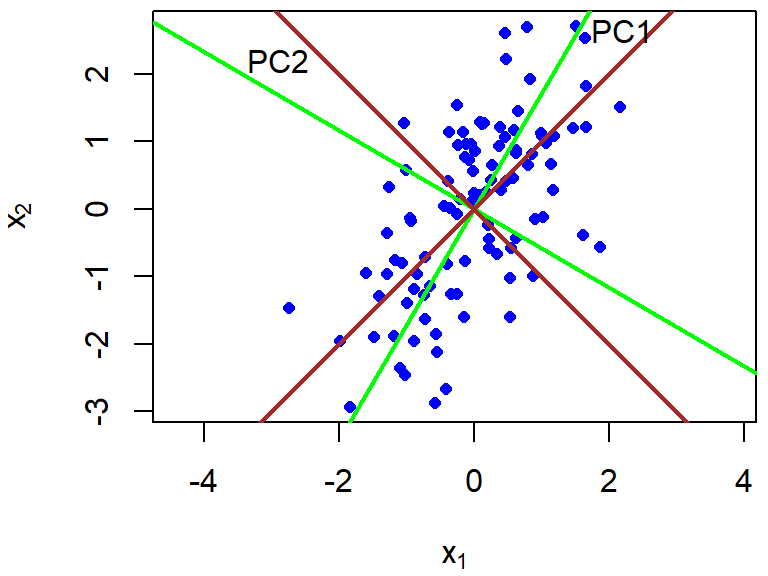Figure 2.4: Principal components of a two dimensional data set based on the covariance matrix (green) and the correlation matrix (brown). The choice between correlation or covariance matrix is essential and important. The covariance matrix is an unstandardized correlation matrix. Therefore, the units, i.e., whether cm or m are used, influence the results of the PCA if it is based on the covariance matrix. When the PCA is based on the covariance matrix, the results will change, when we change the units of one variable, e.g., from cm to m. Basing the PCA on the covariance matrix only makes sense, when the variances are comparable among the variables, i.e., if all variables are measured in the same unit and we would like to weight each variable according to its variance. If this is not the case, the PCA must be based on the correlation matrix. pca <- princomp(cbind(x1,x2)) # PCA based on covariance matrix pca <- princomp(cbind(x1,x2), cor=TRUE) # PCA based on correlation matrix loadings(pca) ## ## Loadings: ## Comp.1 Comp.2 ## x1 0.707 0.707 ## x2 0.707 -0.707 ## ## Comp.1 Comp.2 ## SS loadings 1.0 1.0 ## Proportion Var 0.5 0.5 ## Cumulative Var 0.5 1.0 The loadings measure the correlation of each variable with the principal components. They inform about what aspects of the data each component is measuring. The signs of the loadings are arbitrary, thus we can multiplied them by -1 without changing the PCA. Sometimes this can be handy for describing the meaning of the principal component in a paper. For example, combined the number of hunting licenses, the duration of the hunting period and the number of black grouse cocks that were allowed to be hunted per hunter in a principal component in order to measure hunting pressure. All three variables had a negative loading in the first component, so that high values of the component meant low hunting pressure. Before the subsequent analyses, for which a measure of hunting pressure was of interest, the authors changed the signs of the loadings so that this component measured hunting pressure. The proportion of variance explained by each component is, beside the loadings, an important information. If the first few components explain the main part of the variance, it means that maybe not all $$k$$ variables are necessary to describe the data, or, in other words, the original $$k$$ variables contain a lot of redundant information. # extract the variance captured by each component summary(pca) ## Importance of components: ## Comp.1 Comp.2 ## Standard deviation 1.2679406 0.6263598 ## Proportion of Variance 0.8038367 0.1961633 ## Cumulative Proportion 0.8038367 1.0000000 Ridge regression is similar to doing a PCA within a linear model while components with low variance are shrinked to a higher degree than components with a high variance. ## 2.3 Inferential statistics ### 2.3.1 Uncertainty there is never a “yes-or-no” answer there will always be uncertainty Amrhein (2017)[https://peerj.com/preprints/26857] The decision whether an effect is important or not cannot not be done based on data alone. For a decision we should carefully consider the consequences of each decision, the aims we would like to achieve and the data. Consequences, needs and wishes of different stakeholders can be formally combined with the information in data by using decision theoretical methods. In most data analyses, particularly in basic research and when working on case studies, we normally do not consider consequences of decisions. In these cases, our job is extracting the information of data so that this information later can be used by other scientists, stakeholders and politicians to make decisions. Therefore, statistics is describing pattern in data and quantifying the uncertainty of the described patterns that is due to the fact that the data is just a (small) random sample from the population we would like to know. Quantification of uncertainty is only possible if: 1. the mechanisms that generated the data are known 2. the observations are a random sample from the population of interest Most studies aim at understanding the mechanisms that generated the data, thus they are most likely not known. To overcome that problem, we construct models, e.g. statistical models, that are (strong) abstractions of the data generating process. And we report the model assumptions. All uncertainty measures are conditional on the model we used to analyze the data, i.e., they are only reliable, if the model we used somehow realistically describes the data generating process. Because most statistical models do not describe the data generating process well, the true uncertainty almost always is much higher than the one we report. In order to obtain a random sample from the population under study, a good study design is a prerequisite. To illustrate how inference about a big population is drawn from a small sample, we here use simulated data. The advantage of using simulated data is that the mechanism that generated the data is known. However, in the example, we use different models for simulation and analysis. Imagine there are 300000 PhD students on the world and we would like to know how many statistics courses they have taken before they started their PhD (Fig. 2.5). # simulate the virtual true population set.seed(235325) # set seed for random number generator # simulate fake data of the whole population # using an overdispersed Poisson distribution # There is no need to understand more of this model # at this moment of the course than that this model # produces integer numbers (counts). Poisson models # will be introduced later. statscourses <- rpois(300000, rgamma(300000, 2, 3)) # draw a random sample from the population n <- 12 # sample size y <- sample(statscourses, 12, replace=FALSE)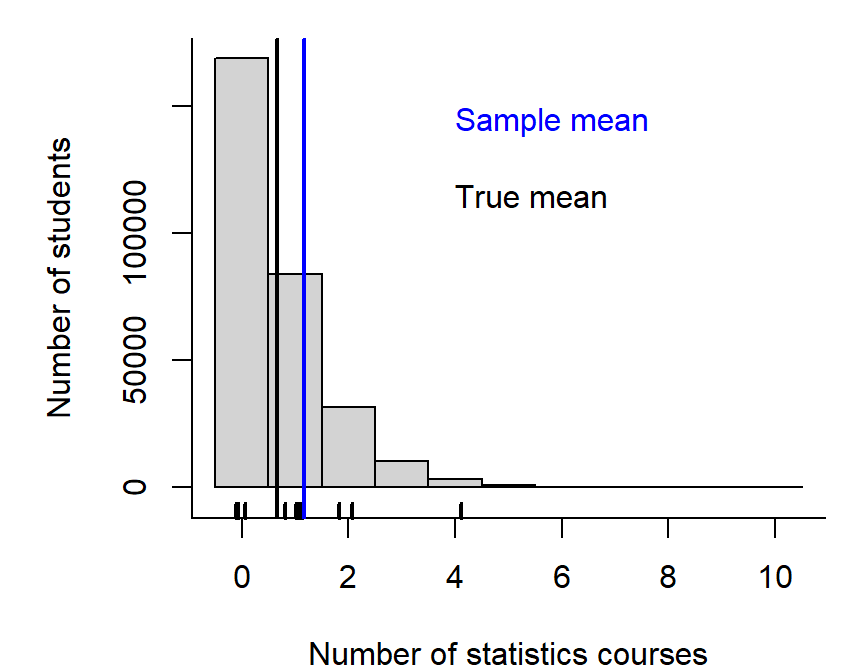Figure 2.5: Histogram of the number of statistics courses 300000 virtual PhD students have taken before their PhD started. The rugs on the x-axis indicate a random sample of the 300000 students. The black vertical line indicates the mean of the 300000 students (true mean) and the blue line indicates the mean of the sample. We observe the sample mean, what do we know about the population mean? There are two different approaches to answer this question. 1) We could ask us, how much the sample mean would scatter, if we repeat the study many times? This approach is called the frequentist statistics. 2) We could ask us for any possible value, what is the probability that it is the true population mean? To do so, we use probability theory and that is called the Bayesian statistics. Both approaches use (essentially similar) models. Only the mathematical techniques to calculate uncertainty measures differ between the two approaches. In cases when beside the data no other information is used to construct the model, then the results are approximately identical (at least for large enough sample sizes). We illustrate what uncertainty intervals mean in Fig. 2.6. A frequentist 95% confidence interval (blue horizontal semgent in Fig. 2.6) is constructed such that, if you were to (hypothetically) repeat the experiment or sampling many many times, 95% of the intervals constructed would contain the true value of the parameter. From the Bayesian posterior distribution we could construct a 95% interval (e.g., by using the 2.5% and 97.5% quantiles). This interval has traditionally been called credible interval. It can be interpreted that we are 95% sure that the true mean is inside that interval. Both interpretations only are reliable if the model is a realistic abstraction of the data generating process (or if the model assumptions are realistic). Because both terms, confidence and credible interval, suggest that the interval indicates confidence or credibility but the intervals actually show uncertainty, it has been suggested to rename the interval into compatibility or uncertainty interval .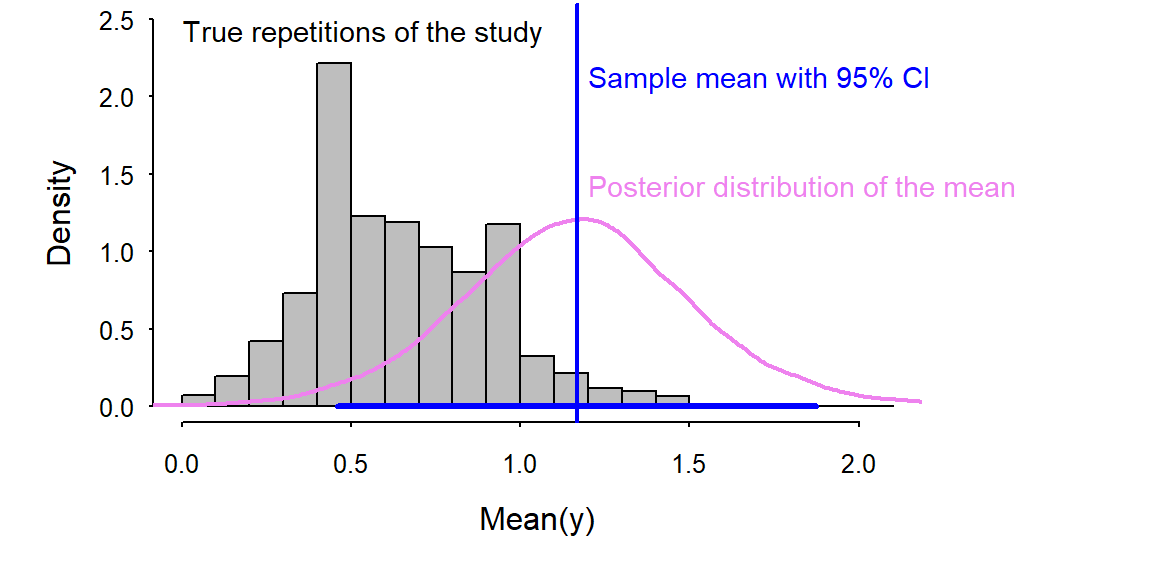Figure 2.6: Histogram of means of repeated samples from the true populations. The scatter of these means visualize the true uncertainty of the mean in this example. The blue vertical line indicates the mean of the original sample. The blue segment shows the 95% confidence interval (obtained by fequensist methods) and the violet line shows the posterior distribution of the mean (obtained by Bayesian methods). For both solutions, we assumed a Normal distribution for the data that is different from the true mechanism that generated the data (which was an overdispersed Poisson model. The star indicates the true mean. ### 2.3.2 Standard error The standard error SE is, beside the uncertainty interval, an alternative possibility to measure uncertainty. It measures an average deviation of the sample mean from the (unknown) true population mean. The frequentist method for obtaining the SE is based on the central limit theorem. According to the central limit theorem the sum of independent, not necessarily normally distributed random numbers are normally distributed when sample size is large enough (Chapter 4). Because the mean is a sum (divided by a constant, the sample size) it can be assumed that the distribution of many means of samples is normal. It can be mathematically shown that the standard error SE equals the standard deviation SD of the sample divided by the square root of the sample size: • frequentist SE = SD/sqrt(n) = $$\frac{\hat{\sigma}}{\sqrt{n}}$$ • Bayesian SE: Using Bayesian methods, the SE is the SD of the posterior distribution. It is very important to keep the difference between SE and SD in mind! SD measures the scatter of the data, whereas SE measures the uncertainty of the mean (or of another estimated parameter, Fig. 2.7). SD is a descriptive statistics describing a characteristics of the data, whereas SE is an inferential statistics showing us how far away we possibly are from the true parameter value. When sample size increases, SE becomes smaller, whereas SD does not change (given the added observations are drawn at random from the same big population as the ones already in the sample).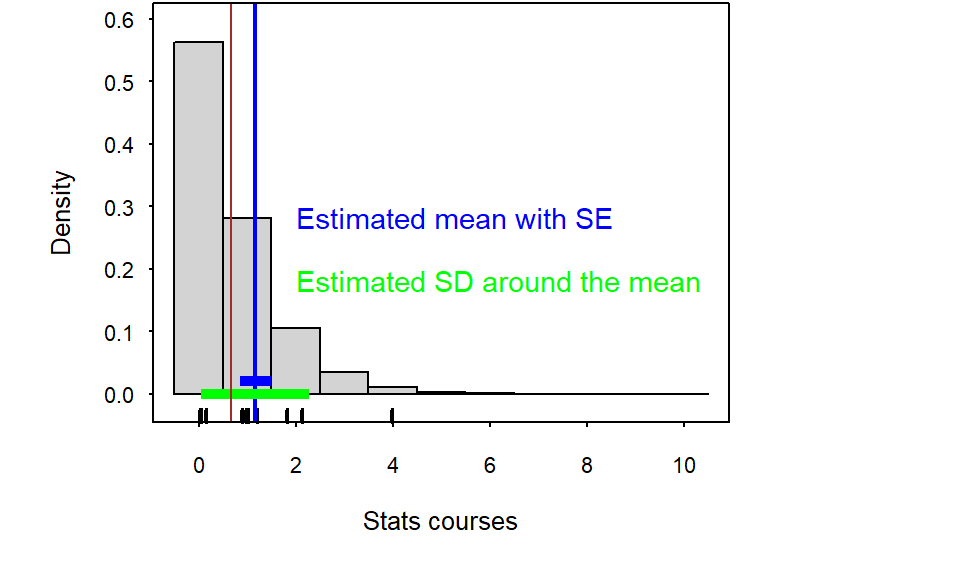Figure 2.7: Illustration of the difference between SD and SE. The SD measures the scatter in the data (for the sample (green tickmarks) in green and for the true population (light grey histogram) in a thin black line. The SE measures the uncertainty of the sample mean (in blue for the sample mean and in bold black for the means of the repeated samples (dark grey histogram)). ## 2.4 Bayes theorem and the common aim of frequentist and Bayesian methods ### 2.4.1 Bayes theorem for discrete events The Bayes theorem describes the probability of event A conditional on event B (the probability of A after B has already occurred) from the probability of B conditional on A and the two probabilities of the events A and B: $$P(A|B) = \frac{P(B|A)P(A)}{P(B)}$$ Imagine, event A is “The person likes wine as a birthday present.” and event B “The person has no car.” The conditional probability of A given B is the probability that a person not owing a car likes wine. Answers from students whether they have a car and what they like as a birthday present are summarized in Table 2.2. Table 2.2: Cross table of the student’s birthday preference and car ownership. car/birthday flowers wine sum no car 6 9 15 car 1 6 7 sum 7 15 22 We can apply the Bayes theorem to obtain the probability that the student likes wine given it has no car, $$P(A|B)$$. Let’s assume that only the ones who prefer wine above flowers among the students go together for having a glass of wine at the bar after the statistics course. While they drink wine, the tell each other about their cars and they obtain the probability that a student has no car given it likes wine, $$P(B|A) = 0.6$$. During the statistics class the teacher asked the students about their car ownership and birthday preference. Therefore, they know that $$P(A) =$$ likes wine $$= 0.68$$ and $$P(B) =$$ no car $$= 0.68$$. With these information, they can obtain the probability that a student likes wine given it has no car, even if not all students without cars went to the bar: $$P(A|B) = \frac{0.6*0.68}{0.68} = 0.6$$. ### 2.4.2 Bayes theorem for continuous parameters When we use the Bayes theorem for analyzing data, then the aim is to make probability statements for parameters. Such a probability statement could be the description of what we know about the population mean after having looked at the data. Because the mean of the population is measured at a continuous scale we use a probability density function to describe what we know about the mean. The Bayes theorem can be formulated for probability density functions denoted with $$p(\theta)$$, e.g. for a parameter $$\theta$$ (for example probability density functions see Chapter 4). What we are interested in is the probability of the parameter $$\theta$$ given the data, i.e., $$p(\theta|y)$$. This probability density function is called the posterior distribution of the parameter $$\theta$$. Here is the Bayes theorem formulated for obtaining the posterior distribution of a parameter from the data $$y$$, the prior distribution of the parameter $$p(\theta)$$ and assuming a model for the data generating process. The data model is defined by the likelihood that specifies how the data $$y$$ is distributed given the parameters $$p(y|\theta)$$: $$p(\theta|y) = \frac{p(y|\theta)p(\theta)}{p(y)} = \frac{p(y|\theta)p(\theta)}{\int p(y|\theta)p(\theta) d\theta}$$ The probability of the data $$p(y)$$ is also called the scaling constant, because it is a constant. It is the integral of the likelihood over all possible values of the parameter(s) of the model. ### 2.4.3 Estimating a mean assuming that the variance is known For illustration, we first describe a simple (unrealistic) example for which it is almost possible to follow the mathematical steps for solving the Bayes theorem even for non-mathematicians. Even if we cannot follow all steps, this example will illustrate the principle how the Bayesian theorem works for continuous parameters. The example is unrealistic because we assume that the variance $$\sigma^2$$ in the data $$y$$ is known. We construct a data model by assuming that $$y$$ is normally distributed: $$p(y|\theta) = Norm(\theta, \sigma)$$, with $$\sigma$$ known. The function $$Norm$$ defines the probability density function of the normal distribution (Chapter 4). The parameter, for which we would like to get the posterior distribution is $$\theta$$, the mean. We specify a prior distribution for $$\theta$$. Because the normal distribution is a conjugate prior for a normal data model with known variance, we use the normal distribution. Conjugate priors have nice mathematical properties (see Chapter 10) and are therefore preferred when the posterior distribution is obtained algebraically. That is the prior: $$p(\theta) = Norm(\mu_0, \tau_0)$$ With the above data, data model and prior, the posterior distribution of the mean $$\theta$$ is defined by: $$p(\theta|y) = Norm(\mu_n, \tau_n)$$, where $$\mu_n= \frac{\frac{1}{\tau_0^2}\mu_0 + \frac{n}{\sigma^2}\bar{y}}{\frac{1}{\tau_0^2}+\frac{n}{\sigma^2}}$$ and $$\frac{1}{\tau_n^2} = \frac{1}{\tau_0^2} + \frac{n}{\sigma^2}$$ $$\bar{y}$$ is the arithmetic mean of the data. Because only this value is needed in order to obtain the posterior distribution, this value is called the sufficient statistics. From the mathematical formulas above and also from Fig. 2.8 we see that the mean of the posterior distribution is a weighted average between the prior mean and $$\bar{y}$$ with weights equal to the precisions ($$\frac{1}{\tau_0^2}$$ and $$\frac{n}{\sigma^2}$$).Figure 2.8: Hypothetical example showing the result of applying the Bayes theorem for obtaining a posterior distribution of a continuous parameter. The likelhood is defined by the data and the model, the prior is expressing the knowledge about the parameter before looking at the data. Combining the two distributions using the Bayes theorem results in the posterior distribution. ### 2.4.4 Estimating the mean and the variance We now move to a more realistic example, which is estimating the mean and the variance of a sample of weights of Snowfinches Montifringilla nivalis (Fig. 2.9). To analyze those data, a model with two parameters (the mean and the variance or standard deviation) is needed. The data model (or likelihood) is specified as $$p(y|\theta, \sigma) = Norm(\theta, \sigma)$$.Figure 2.9: Snowfinches stay above the treeline for winter. They come to feeders. # weight (g) y <- c(47.5, 43, 43, 44, 48.5, 37.5, 41.5, 45.5) n <- length(y) Because there are two parameters, we need to specify a two-dimensional prior distribution. We looked up in that the conjugate prior distribution in our case is an Normal-Inverse-Chisquare distribution: $$p(\theta, \sigma) = N-Inv-\chi^2(\mu_0, \sigma_0^2/\kappa_0; v_0, \sigma_0^2)$$ From the same reference we looked up how the posterior distribution looks like in our case: $$p(\theta,\sigma|y) = \frac{p(y|\theta, \sigma)p(\theta, \sigma)}{p(y)} = N-Inv-\chi^2(\mu_n, \sigma_n^2/\kappa_n; v_n, \sigma_n^2)$$, with $$\mu_n= \frac{\kappa_0}{\kappa_0+n}\mu_0 + \frac{n}{\kappa_0+n}\bar{y}$$, $$\kappa_n = \kappa_0+n$$, $$v_n = v_0 +n$$, $$v_n\sigma_n^2=v_0\sigma_0^2+(n-1)s^2+\frac{\kappa_0n}{\kappa_0+n}(\bar{y}-\mu_0)^2$$ For this example, we need the arithmetic mean $$\bar{y}$$ and standard deviation $$s^2$$ from the sample for obtaining the posterior distribution. Therefore, these two statistics are the sufficient statistics. The above formula look intimidating, but we never really do that calculations. We let Rdoing that for us in most cases by simulating many numbers from the posterior distribution. In the end, we can visualize the distribution of these many numbers to have a look at the posterior distribution. In Fig. 2.10 the two-dimensional $$(\theta, \sigma)$$ posterior distribution is visualized by using simulated values. The two dimensional distribution is called the joint posterior distribution. The mountain of dots in Fig. 2.10 visualize the Normal-Inverse-Chisquare that we calculated above. When all values of one parameter is displayed in a histogram ignoring the values of the other parameter, it is called the marginal posterior distribution. Algebraically, the marginal distribution is obtained by integrating one of the two parameters out over joint posterior distribution. This step is definitively way easier when simulated values from the posterior distribution are available! For the Snowfinch weights example, with a normal data model and a conjugate prior the marginal posterior distribution of the mean $$\theta$$ becomes a t-distribution and the one of $$\sigma$$ a inverse-Chisquare distribution. Another nice property of the posterior distribution in our example is that a horizontal section through the joint posterior distribution (at a fixed value of $$\sigma$$, i.e. a fixed variance) leads to a normal distribution. In other words, the density of the dots in the plot at a fixed value of $$\sigma$$ follows a normal density function.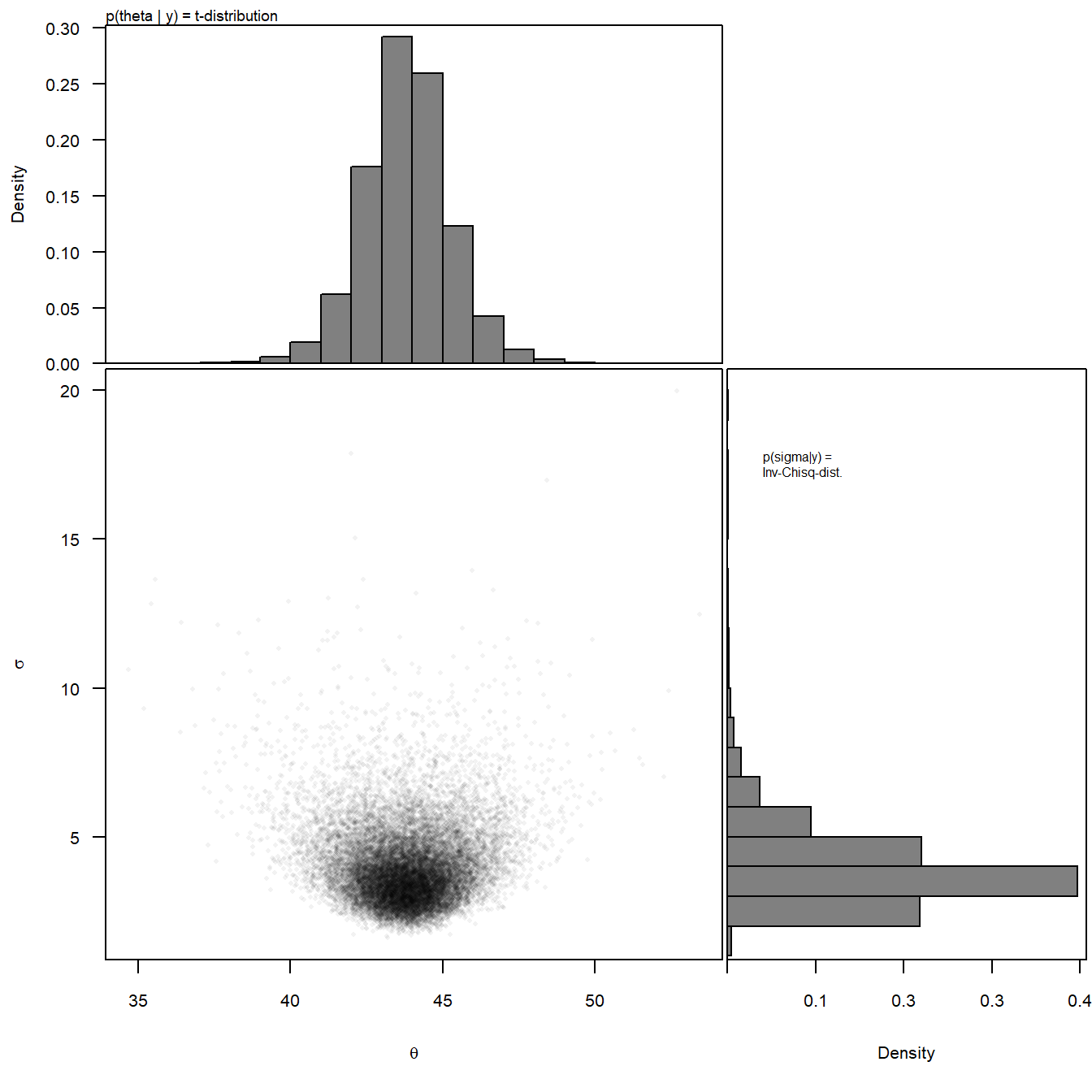Figure 2.10: Visualization of the joint posterior distribution for the mean and standard deviation of Snowfinch weights. The lower left panel shows the two-dimensional joint posterior distribution, whereas the upper and right panel show the marginal posterior distributions of each parameter separately. The marginal posterior distributions of every parameter is what we normally report in a paper. They inform us about what we know about these parameters (of the big unmeasured population) after having looked at the data. In our example the the marginal distribution of the mean is a t-distribution (Chapter 4). Frequentist statistical methods also use a t-distribution to describe the uncertainty of a mean for the case when the variance is not known. Thus, frequentist methods came to the same solution using a completely different approach and different techniques. Doesn’t that increase dramatically our trust in statistical methods? ## 2.5 Classical frequentist tests and alternatives ### 2.5.1 Nullhypothesis testing Null hypothesis testing is constructing a model that describes how the data would look like in case of what we expect to be would not be. Then, the data is compared to how the model thinks the data should look like. If the data does not look like the model thinks they should, we reject that model and accept that our expectation may be true. To decide whether the data looks like the model constructed from the null hypothesis thinks the data should look like the p-value is used. The p-value is the probability of observing the data or more extreme data given the null hypothesis is true. Small p-values indicate that it is rather unlikely to observe the data or more extreme data given the null hypothesis $$H_0$$ is true. Null hypothesis testing is problematic. We discuss some of the problems after having introduces the most commonly used classical tests. ### 2.5.2 Comparison of a sample with a fixed values (one-sample t-test) A null hypothesis is used to construct a model that can generate (hypothetical) data. For example, a null hypothesis could be $$H_0:$$“The mean of Snowfinch weights is exactly 40g.” A normal distribution with a mean of $$\mu_0=40$$ and a variance equal to the estimated variance in the data $$s^2$$ is then assumed to describe how we would expect the data to look like given the null hypothesis was true. From that model it is possible to calculate the distribution of hypothetical means of many different hypothetical samples of sample size $$n$$. The result is a t-distribution (Fig. 2.11). In classical tests, the distribution is standardized so that its variance was one. Then the sample mean, or in classical tests a standardized difference between the mean and the hypothetical mean of the null hypothesis (here 40g), called test statistics $$t = \frac{\bar{y}-\mu_0}{\frac{s}{\sqrt{n}}}$$, is compared to that (standardized) t-distribution. If the test statistics falls well within the expected distribution the null hypothesis is accepted. Then, the data is well compatible with the null hypothesis. However, if the test statistics falls in the tails or outside the distribution, then the null hypothesis is rejected and we could write that the mean weight of Snowfinches is statistically significantly different from 40g. Unfortunately, we cannot infer about the probability of the null hypothesis and how relevant the result is.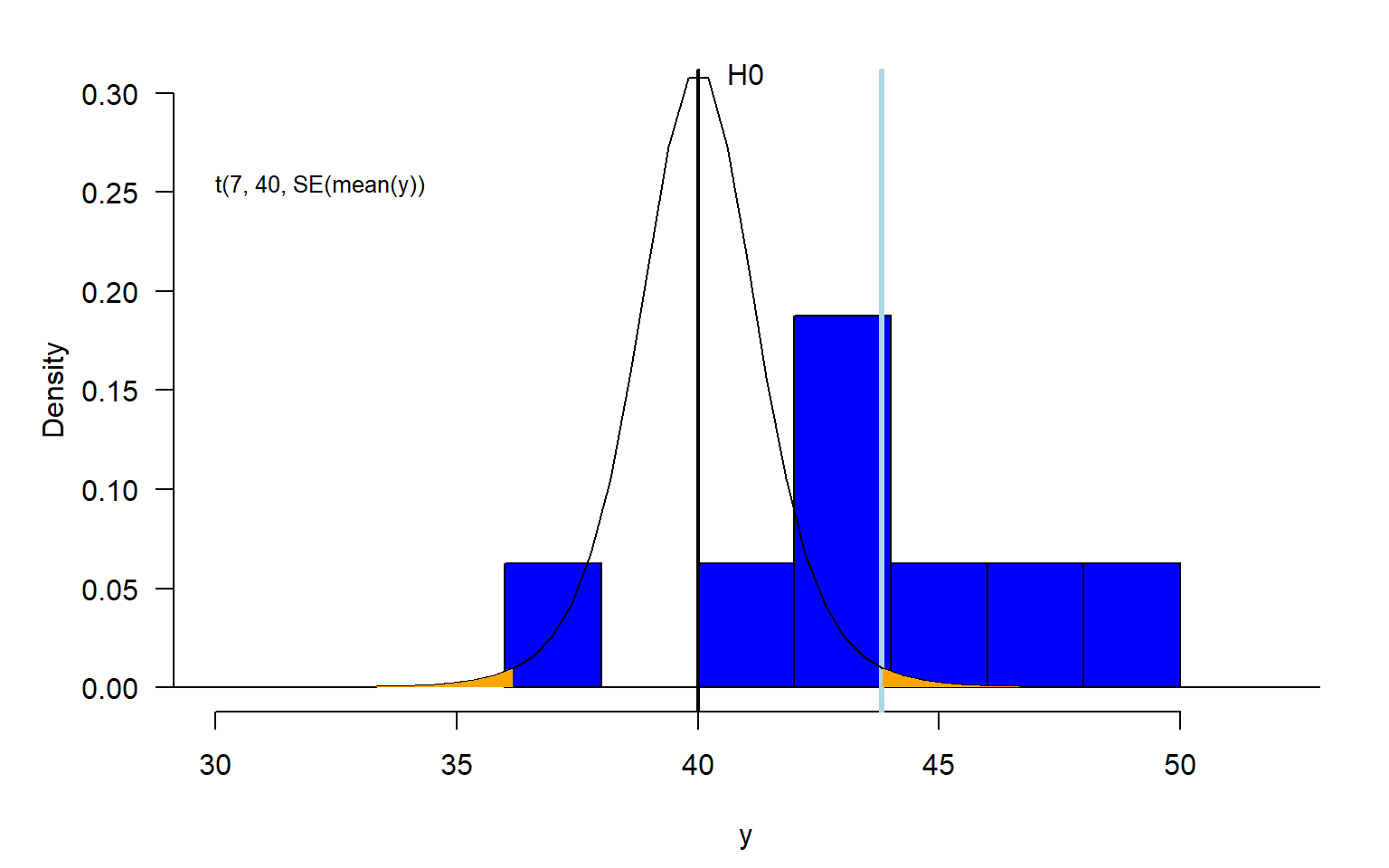Figure 2.11: Illustration of a one-sample t-test. The blue histogram shows the distribution of the measured weights with the sample mean (lightblue) indicated as a vertical line. The black line is the t-distribution that shows how hypothetical sample means are expected to be distributed if the big population of Snowfinches has a mean weight of 40g (i.e., if the null hypothesis was true). Orange area shows the area of the t-distribution that lays equal or farther away from 40g than the sample mean. The orange area is the p-value. We can use the r-function t.test to calculate the p-value of a one sample t-test. t.test(y, mu=40) ## ## One Sample t-test ## ## data: y ## t = 3.0951, df = 7, p-value = 0.01744 ## alternative hypothesis: true mean is not equal to 40 ## 95 percent confidence interval: ## 40.89979 46.72521 ## sample estimates: ## mean of x ## 43.8125 The output of the r-function t.test also includes the mean and the 95% confidence interval (or compatibility or uncertainty interval) of the mean. The CI could, alternatively, be obtained as the 2.5% and 97.5% quantiles of a t-distribution with a mean equal to the sample mean, degrees of freedom equal to the sample size minus one and a standard deviation equal to the standard error of the mean. # lower limit of 95% CI mean(y) + qt(0.025, df=length(y)-1)*sd(y)/sqrt(n)  ##  40.89979 # upper limit of 95% CI mean(y) + qt(0.975, df=length(y)-1)*sd(y)/sqrt(n)  ##  46.72521 When applying the Bayes theorem for obtaining the posterior distribution of the mean we end up with the same t-distribution as described above, in case we use flat prior distributions for the mean and the standard deviation. Thus, the two different approaches end up with the same result! par(mar=c(4.5, 5, 2, 2)) hist(y, col="blue", xlim=c(30,52), las=1, freq=FALSE, main=NA, ylim=c(0, 0.3)) abline(v=mean(y), lwd=2, col="lightblue") abline(v=40, lwd=2) lines(density(bsim@coef)) text(45, 0.3, "posterior distribution\nof the mean of y", cex=0.8, adj=c(0,1), xpd=NA)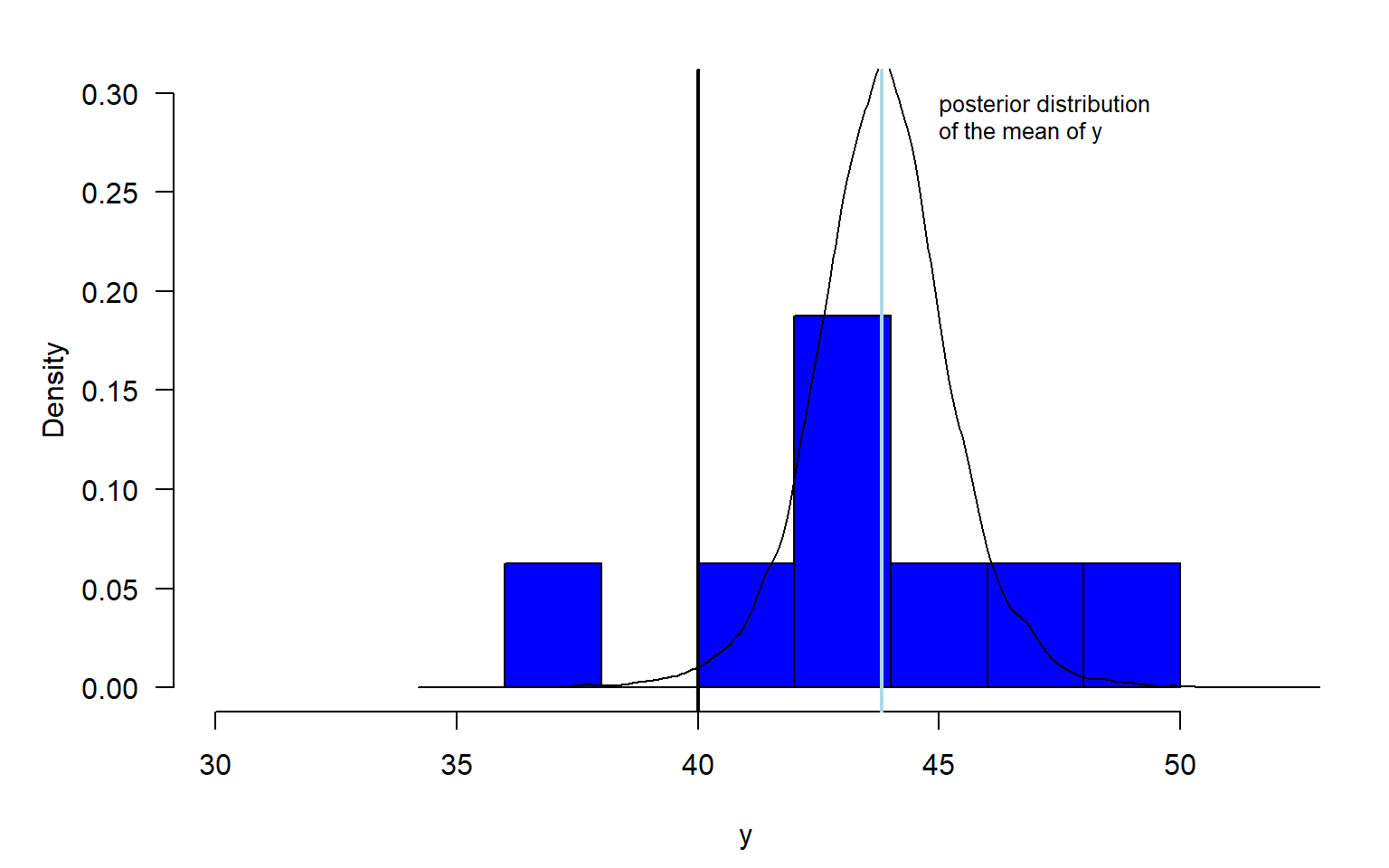Figure 2.12: Illustration of the posterior distribution of the mean. The blue histogram shows the distribution of the measured weights with the sample mean (lightblue) indicated as a vertical line. The black line is the posterior distribution that shows what we know about the mean after having looked at the data. The area under the posterior density function that is larger than 40 is the posterior probability of the hypothesis that the true mean Snwofinch weight is larger than 40g. The posterior probability of the hypothesis that the true mean Snowfinch weight is larger than 40g, $$P(H:\mu>40) =$$, is equal to the proportion of simulated random values from the posterior distribution, saved in the vector bsim@coef, that are larger than 40. # Two ways of calculating the proportion of values # larger than a specific value within a vector of values round(sum(bsim@coef[,1]>40)/nrow(bsim@coef),2) ##  0.99 round(mean(bsim@coef[,1]>40),2) ##  0.99 # Note: logical values TRUE and FALSE become # the numeric values 1 and 0 within the functions sum() and mean() We, thus, can be pretty sure that the mean Snowfinch weight (in the big world population) is larger than 40g. Such a conclusion is not very informative, because it does not tell us how much larger we can expect the mean Snowfinch weight to be. Therefore, we prefer reporting a credible interval (or compatibility interval or uncertainty interval) that tells us what values for the mean Snowfinch weight are compatible with the data (given the data model we used realistically reflects the data generating process). Based on such an interval, we can conclude that we are pretty sure that the mean Snowfinch weight is between 40 and 48g. # 80% credible interval, compatibility interval, uncertainty interval quantile(bsim@coef[,1], probs=c(0.1, 0.9)) ## 10% 90% ## 42.07725 45.54080 # 95% credible interval, compatibility interval, uncertainty interval quantile(bsim@coef[,1], probs=c(0.025, 0.975)) ## 2.5% 97.5% ## 40.90717 46.69152 # 99% credible interval, compatibility interval, uncertainty interval quantile(bsim@coef[,1], probs=c(0.005, 0.995)) ## 0.5% 99.5% ## 39.66181 48.10269 ### 2.5.3 Comparison of the locations between two groups (two-sample t-test) Many research questions aim at measuring differences between groups. For example, we could be curious to know how different in size car owner are from people not owning a car. A boxplot is a nice possibility to visualize the ell length measurements of two (or more) groups (Fig. 2.13). From the boxplot, we do not see how many observations are in the two samples. We can add that information to the plot. The boxplot visualizes the samples but it does not show what we know about the big (unmeasured) population mean. To show that, we need to add a compatibility interval (or uncertainty interval, credible interval, confidence interval, in brown in Fig. 2.13).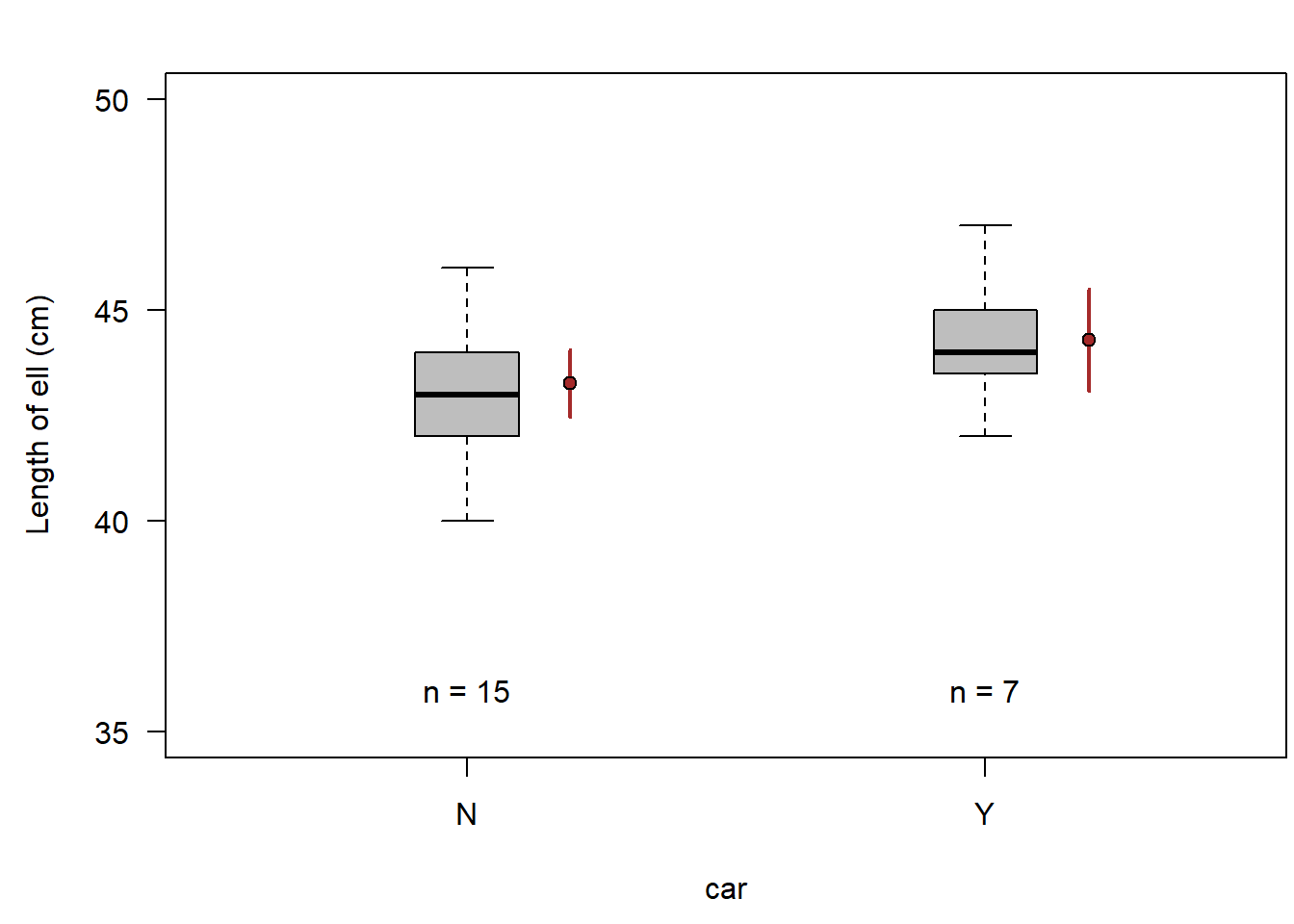Figure 2.13: Ell length of car owners (Y) and people not owning a car (N). Horizontal bar = median, box = interquartile range, whiskers = extremest observation within 1.5 times the interquartile range from the quartile, circles=observations farther than 1.5 times the interquartile range from the quartile. Filled brown circles = means, vertical brown bars = 95% compatibility interval. When we added the two means with a compatibility interval, we see what we know about the two means, but we do still not see what we know about the difference between the two means. The uncertainties of the means do not show the uncertainty of the difference between the means. To do so, we need to extract the difference between the two means from a model that describes (abstractly) how the data has been generated. Such a model is a linear model that we will introduce in Chapter 11. The second parameter measures the differences in the means of the two groups. And from the simulated posterior distribution we can extract a 95% compatibility interval. Thus, we can conclude that the average ell length of car owners is with high probability between 0.5 cm smaller and 2.5 cm larger than the averag ell of people not having a car. mod <- lm(ell~car, data=dat) mod ## ## Call: ## lm(formula = ell ~ car, data = dat) ## ## Coefficients: ## (Intercept) carY ## 43.267 1.019 bsim <- sim(mod, n.sim=nsim) quantile(bsim@coef[,"carY"], prob=c(0.025, 0.5, 0.975)) ## 2.5% 50% 97.5% ## -0.501348 1.014478 2.494324 The corresponding two-sample t-test gives a p-value for the null hypothesis: “The difference between the two means equals zero.” a confidence interval for the difference and the two means. While the function lmgives the difference Y minus N, the function t.testgives the difference N minus Y. Luckily the two means are also given in the output, so we know which group mean is the larger one. t.test(ell~car, data=dat, var.equal=TRUE) ## ## Two Sample t-test ## ## data: ell by car ## t = -1.4317, df = 20, p-value = 0.1677 ## alternative hypothesis: true difference in means between group N and group Y is not equal to 0 ## 95 percent confidence interval: ## -2.5038207 0.4657255 ## sample estimates: ## mean in group N mean in group Y ## 43.26667 44.28571 In both possibilities, we used to compare the to means, the Bayesian posterior distribution of the difference and the t-test or the confidence interval of the difference, we used a data model. We thus assumed that the observations are normally distributed. In some cases, such an assumption is not a reasonable assumption. Then the result is not reliable. In such cases, we can either search for a more realistic model or use non-parametric (also called distribution free) methods. Nowadays, we have almost infinite possibilities to construct data models (e.g. generalized linear models and beyond). Therefore, we normally start looking for a model that fits the data better. However, in former days, all these possiblities did not exist (or were not easily available for non-mathematicians). Therefore, we here introduce two of such non-parametric methods, the Wilcoxon-test (or Mann-Whitney-U-test) and the randomisation test. Some of the distribution free statistical methods are based on the rank instead of the value of the observations. The principle of the Wilcoxon-test is to rank the observations and sum the ranks per group. It is not completely true that the non-parametric methods do not have a model. The model of the Wilcoxon-test “knows” how the difference in the sum of the ranks between two groups is distributed given the mean of the two groups do not differ (null hypothesis). Therefore, it is possible to get a p-value, e.g. by the function wilcox.test. wilcox.test(ell~car, data=dat) ## ## Wilcoxon rank sum test with continuity correction ## ## data: ell by car ## W = 34.5, p-value = 0.2075 ## alternative hypothesis: true location shift is not equal to 0 The note in the output tells us that ranking is ambiguous, when some values are equal. Equal values are called ties when they should be ranked. The result of the Wilcoxon-test tells us how probable it is to observe the difference in the rank sum between the two sample or a more extreme difference given the means of the two groups are equal. That is at least something. A similar result is obtained by using a randomisation test. This test is not based on ranks but on the original values. The aim of the randomisation is to simulate a distribution of the difference in the arithmetic mean between the two groups assuming this difference would be zero. To do so, the observed values are randomly distributed among the two groups. Because of the random distribution among the two groups, we expect that, if we repeat that virtual experiment many times, the average difference between the group means would be zero (both virtual samples are drawn from the same big population). We can use a loop in R for repeating the random re-assignement to the two groups and, each time, extracting the difference between the group means. As a result, we have a vector of many (nsim) values that all are possible differences between group means given the two samples were drawn from the same population. The proportion of these values that have an equal or larger absolute value give the probability that the observed or a larger difference between the group means is observed given the null hypothesis would be true, thus that is a p-value. diffH0 <- numeric(nsim) for(i in 1:nsim){ randomcars <- sample(dat$car)
rmod <- lm(ell~randomcars, data=dat)
diffH0[i] <- coef(rmod)["randomcarsY"]
}
mean(abs(diffH0)>abs(coef(mod)["carY"])) # p-value
##  0.1858

Visualizing the possible differences between the group means given the null hypothesis was true shows that the observed difference is well within what is expected if the two groups would not differ in their means (Fig. 2.14).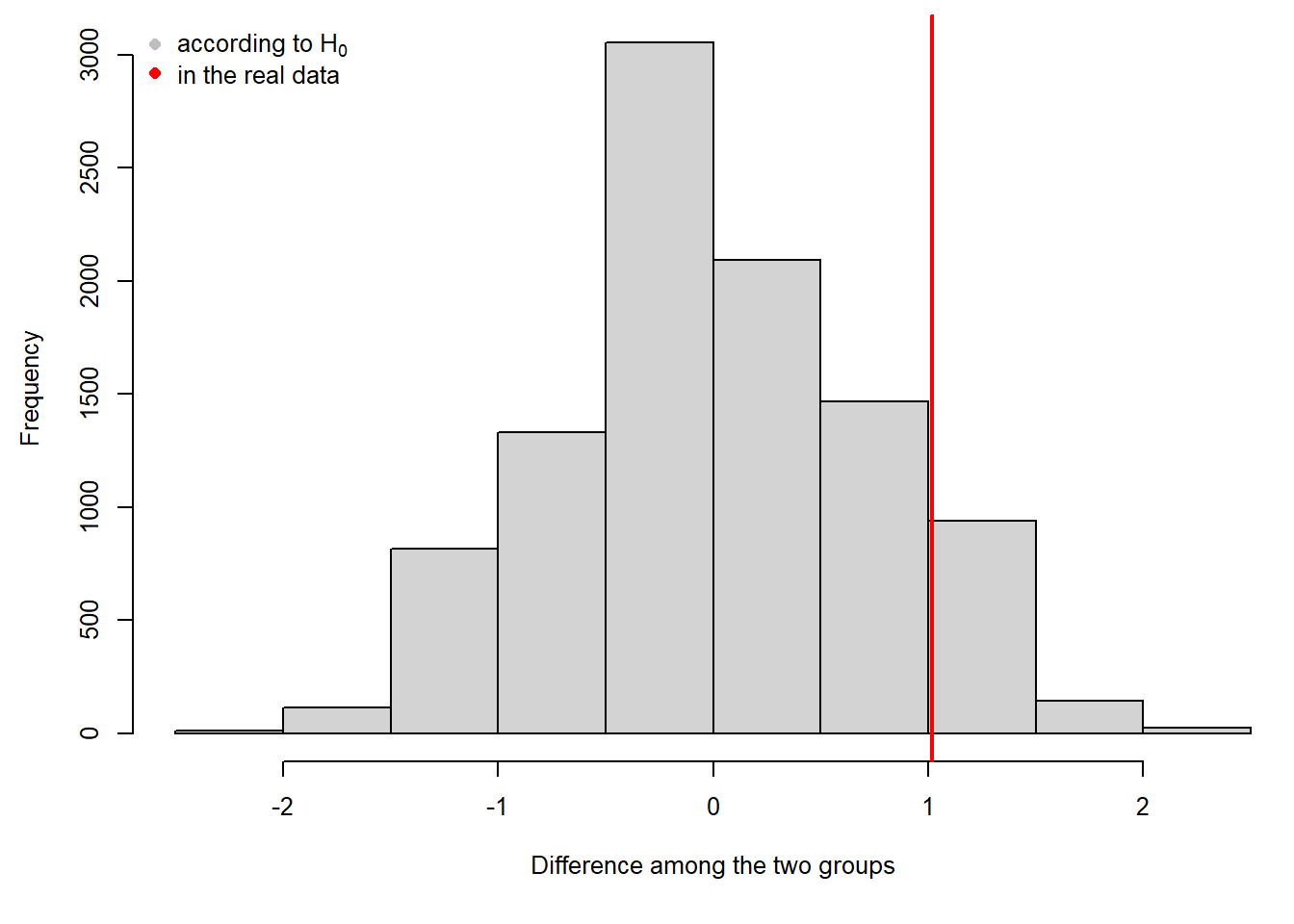Figure 2.14: Histogram if differences between the means of randomly assigned groups (grey) and the difference between the means of the two observed groups (red)

The randomization test results in a p-value and, we could also report the observed difference between the group means. However, it does not tell us, what values of the difference all would be compatible with the data. We do not get an uncertainty measurement for the difference.

In order to get a compatibility interval without assuming a distribution for the data (thus non-parametric) we could bootstrap the samples.

Bootstrapping is sampling observations from the data with replacement. For example, if we have a sample of 8 observations, we draw 8 times a random observation from the 8 observation. Each time, we assume that all 8 observations are available. Thus a bootstrapped sample could include some observations several times, whereas others are missing. In this way, we simulate the variance in the data that is due to the fact that our data do not contain the whole big population.

Also bootstrapping can be programmed in R using a loop.

diffboot <- numeric(1000)
for(i in 1:nsim){
ngroups <- 1
while(ngroups==1){
bootrows <- sample(1:nrow(dat), replace=TRUE)
ngroups <- length(unique(dat\$car[bootrows]))
}
rmod <- lm(ell~car, data=dat[bootrows,])
diffboot[i] <- coef(rmod)
}
quantile(diffboot, prob=c(0.025, 0.975))
##       2.5%      97.5%
## -0.3395643  2.4273810

The resulting values for the difference between the two group means can be interpreted as the distribution of those differences, if we had repeated the study many times (Fig. 2.15). A 95% interval of the distribution corresponds to a 95% compatibility interval (or confidence interval or uncertainty interval).

hist(diffboot); abline(v=coef(mod), lwd=2, col="red")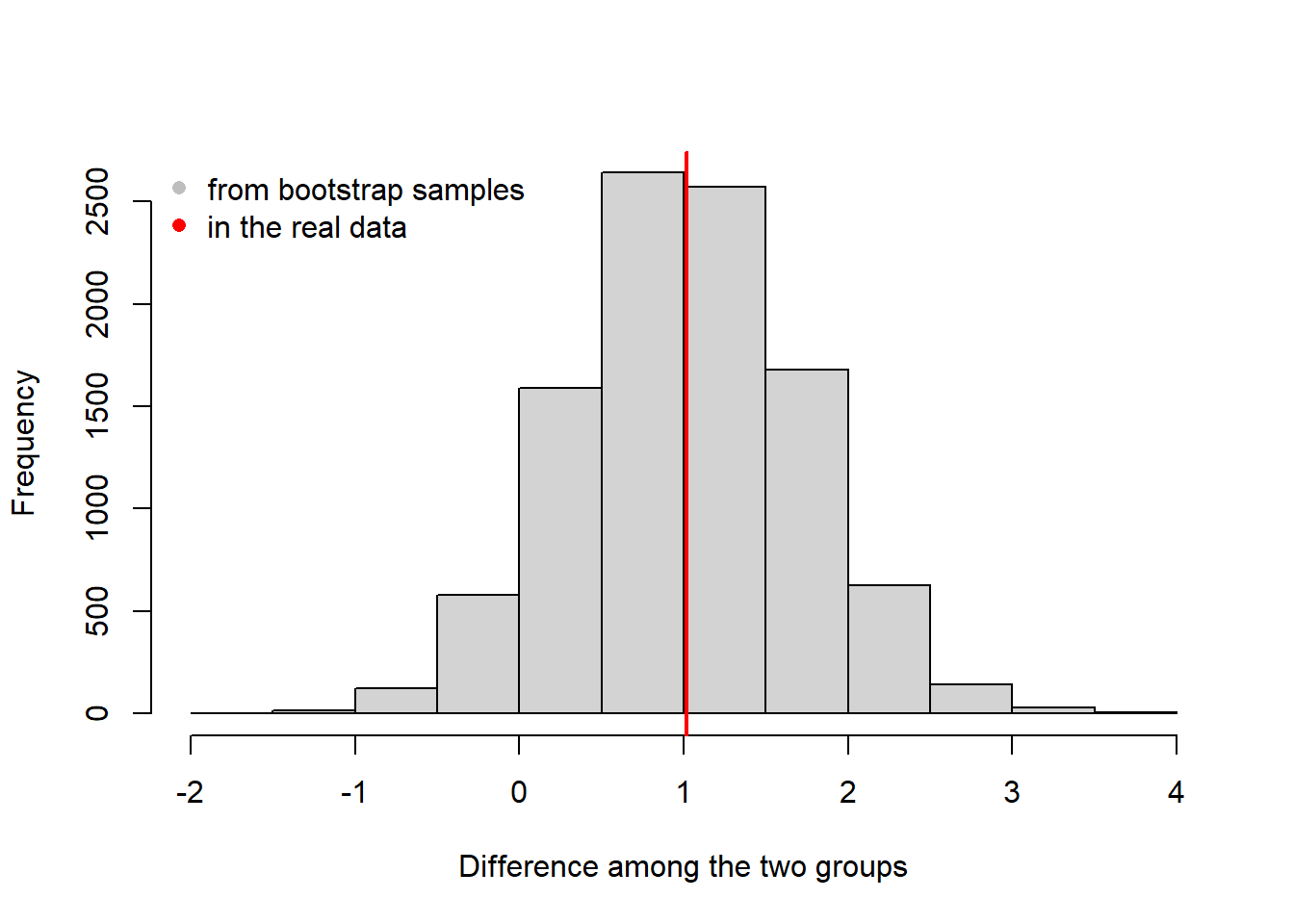Figure 2.15: Histogram of the boostrapped differences between the group means (grey) and the observed difference.

For both methods, randomisation test and bootstrapping, we have to assume that all observations are independent. Randomization and bootstrapping becomes complicated or even unfeasible when data are structured.

## 2.6 Summary

Bayesian data analysis is applying the Bayes theorem for summarizing knowledge based on data, priors and the model assumptions.

Frequentist statistics is quantifying uncertainty by hypothetical repetitions.